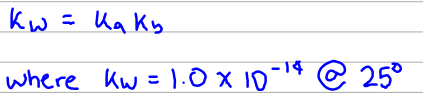# Problem: The Ka of HCN is 6.2 x 10 -10. What is the Kb value for CN - at 25°C

###### FREE Expert Solution
99% (165 ratings)
###### FREE Expert Solution

Since CN-  is the conjugate base of the weak acid HCN, we can relate Ka and Kb by the equation below:Take note that the Kw at room temperature is something that you must memorize.

99% (165 ratings)###### Problem Details

The Ka of HCN is 6.2 x 10 -10. What is the Kb value for CN - at 25°C

What scientific concept do you need to know in order to solve this problem?

Our tutors have indicated that to solve this problem you will need to apply the Ka and Kb concept. You can view video lessons to learn Ka and Kb. Or if you need more Ka and Kb practice, you can also practice Ka and Kb practice problems.

What is the difficulty of this problem?

Our tutors rated the difficulty ofThe Ka of HCN is 6.2 x 10 -10. What is the Kb value for CN -...as low difficulty.

How long does this problem take to solve?

Our expert Chemistry tutor, Sabrina took 1 minute and 28 seconds to solve this problem. You can follow their steps in the video explanation above.

What professor is this problem relevant for?

Based on our data, we think this problem is relevant for Professor Golde's class at PITT.